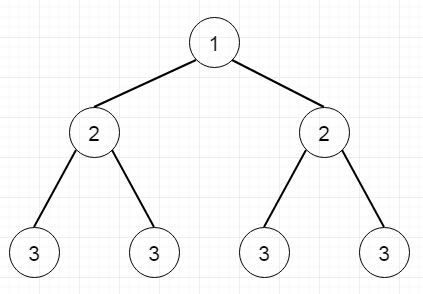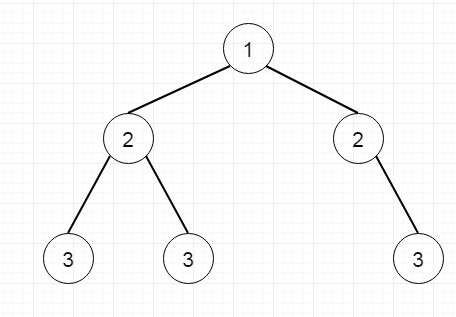# Program to check whether given tree is symmetric tree or not in Python

Suppose we have one binary tree. We have to check whether the tree is symmetric tree or not. A tree will be said to be symmetric if it is same when we take the mirror image of it. From these two trees, the first one is symmetric, but second one is not.To solve this, we will follow these steps.

• We will call following steps recursively. The function will be solve(root, root)

• if the node1 and node2 are empty, then return true

• if either node1 or node2 is empty, then return false

• return true when node1.val = node2.val and solve(node1.left, node2.right) and solve(node1.right, node2.left)

Let us see the following implementation to get better understanding −

## Example

Live Demo

class TreeNode:
def __init__(self, data, left = None, right = None):
self.data = data
self.left = left
self.right = right
class Solution(object):
def isSymmetric(self, root):
return self.solve(root,root)
def solve(self,node1,node2):
if not node1 and not node2:
return True
if not node1 or not node2:
return False
return node1.data == node2.data and
self.solve(node1.left,node2.right) and
self.solve(node1.right,node2.left)
root = TreeNode(1)
root.left = TreeNode(2)
root.right = TreeNode(2)
root.left.left = TreeNode(3)
root.left.right = TreeNode(4)
root.right.left = TreeNode(4)
root.right.right = TreeNode(3)
ob1 = Solution()
print(ob1.isSymmetric(root))

## Input

root = TreeNode(1)
root.left = TreeNode(2)
root.right = TreeNode(2)
root.left.left = TreeNode(3)
root.left.right = TreeNode(4)
root.right.left = TreeNode(4)
root.right.right = TreeNode(3)

## Output

True